# ACT Math : How to find if of acute / obtuse isosceles triangle are similar

## Example Questions

### Example Question #219 : Plane Geometry

Triangle A and Triangle B are similar isosceles triangles. Triangle A's sides measure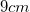, and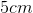. Two of the angles in Triangle A each measure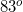. Triangle B's sides measure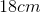, and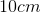. What is the measure of the smallest angle in Triangle B?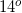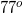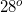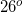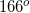Explanation:

Because the interior angles of a triangle add up to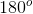, and two of Triangle A's interior angles measure, we must simply add the two given angles and subtract fromto find the missing angle: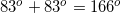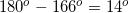Therefore, the missing angle (and the smallest) from Triangle A measures. If the two triangles are similar, their interior angles must be congruent, meaning that the smallest angle is Triangle B is also.

The side measurements presented in the question are not needed to find the answer!

### Example Question #220 : Plane Geometry

Triangle A and Triangle B are similar isosceles triangles. Triangle A has a base of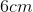and a height of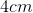. Triangle B has a base of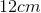. What is the length of Triangle B's two congruent sides?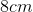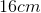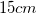Explanation:

We must first find the length of the congruent sides in Triangle A. We do this by setting up a right triangle with the base and the height, and using the Pythagorean Theorem to solve for the missing side (). Because the height line cuts the base in half, however, we must use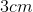for the length of the base's side in the equation instead of. This is illustrated in the figure below: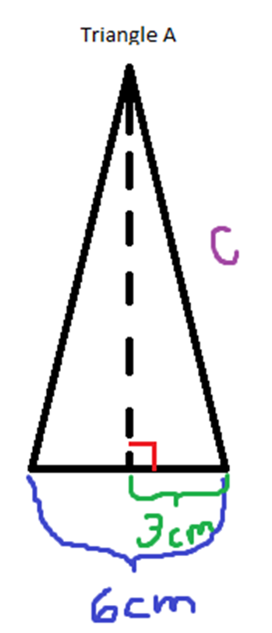Using the base ofand the height of, we use the Pythagorean Theorem to solve for: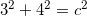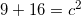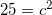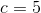Therefore, the two congruent sides of Triangle A measure; however, the question asks for the two congruent sides of Triangle B. In similar triangles, the ratio of the corresponding sides must be equal. We know that the base of Triangle A isand the base of Triangle B is. We then set up a cross-multiplication using the ratio of the two bases and the ratio ofto the side we're trying to find (), as follows: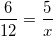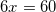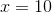Therefore, the length of the congruent sides of Triangle B is.

### Example Question #221 : Plane Geometry

Isosceles triangles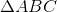and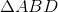share common side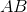is an obtuse triangle with sides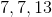is also an obtuse isosceles triangle, where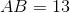. What is the measure of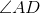?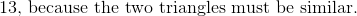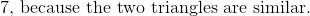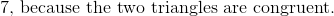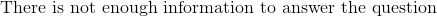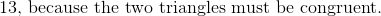In order to prove triangle congruence, the triangles must have SAS, SSS, AAS, or ASA congruence. Here, we have one common side (S), and no other demonstrated congruence. Hence, we cannot guarantee that sideis not one of the two congruent sides of, so we cannot state congruence with.